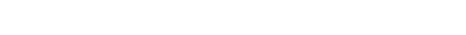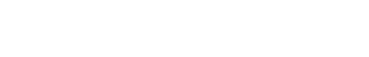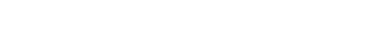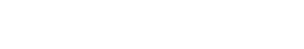# Crackmes.de – gama’s mndg

This crackme is rated Difficulty: 2 – Needs a little brain (or luck). Although it is an easier crackme and it was published almost half a year ago, there are no accepted solutions yet. There are many comments for this crackme with people who solved this crackme, however, they all used some kind of brute forcing or even patching. This solutions show how to generate serials mathematically. If you run the crackme without debugger and enter an invalid serial, you get the dialog box shown in the following screenshot:If you run the crackme with a debugger like OllyDbg (or attach to the running process), entering an invalid serial will most likely lead to a memory access violation similar to this:This crackme uses Anti-Debugging techniques. The first part of this solution discusses the two anti-debugging tricks of this crackme and how to remove those check with patches.

## Anti-Debugging

Finding the subroutine that checks the serial is trivial: search for the string “Sorry, the serial is invalid :(” and you get the following clean code sequence:The subroutine at dword_405000 – I renamed it to validate_serial- is clearly calculating a value based on the serial. If the return value of validate_serial is 0xB528B18B, then the serial is valid, otherwise it is invalid. The subroutine validate_serial is located at an unusual place:

.data:00405000 validate_serial dd 8000000h


Offset 0x80000000 is far from the image base and likely created during runtime. Here is the disassembly of validate_serial when it is called first:

debug138:08000000 push    edx
debug138:08000001 mov     al, [ebp+25h]
debug138:08000004 or      dword_101BFEC[ebx], edi
debug138:0800000A jmp     far ptr 4E0h:0C0D08815h


This doesn’t look like valid code, and will probably lead to the memory access violation exceptions shown before. If we would have run the crackme without debugger, then offset 0x80000000 probably looks different. First, lets find the code location that creates and writes offset 0x8000000. We find the relevant code segment by looking for tale-tale API calls to VirtualAlloc with starting address 0x80000000. Here is the code snippet that allocates the memory and probably writes to it in sub_4015A0:

.text:00401633                 push    40h             ; flProtect
.text:00401635                 push    3000h           ; flAllocationType
.text:0040163A                 push    10000h          ; dwSize
.text:00401644                 call    VirtualAlloc
.text:0040164A                 call    sub_4015A0
.text:0040164F                 mov     [ebp+var_82B], al
.text:00401655                 xor     esi, esi
.text:00401657                 jmp     short loc_40167D


Let’s dive into sub_4015A0 and look for anti-debugging measures.

### OllyDbg.exe

Inside sub_4015A0, we find the following loop:

.text:004015A0 sub_4015A0      proc near               ; CODE XREF: DialogFunc+6Ap
.text:004015A0
.text:004015A0 olly_dbg        = byte ptr -0Ch
.text:004015A0 var_1           = byte ptr -1
.text:004015A0
.text:004015A0                 push    ebp
.text:004015A1                 mov     ebp, esp
.text:004015A3                 sub     esp, 0Ch
.text:004015A6                 lea     ecx, [ebp+olly_dbg]
.text:004015A9                 mov     edx, offset unk_404074
.text:004015AE                 mov     eax, [edx]
.text:004015B0                 mov     [ecx], eax
.text:004015B2                 mov     eax, [edx+4]
.text:004015B5                 mov     [ecx+4], eax
.text:004015B8                 mov     eax, [edx+8]
.text:004015BB                 mov     [ecx+8], eax
.text:004015BE                 xor     eax, eax
.text:004015C0                 jmp     short loc_4015C8
.text:004015C2 ; ---------------------------------------------------------------------------
.text:004015C2
.text:004015C2 loc_4015C2:                             ; CODE XREF: sub_4015A0+2Bj
.text:004015C2                 xor     [ebp+eax+olly_dbg], 81h
.text:004015C7                 inc     eax
.text:004015C8
.text:004015C8 loc_4015C8:                             ; CODE XREF: sub_4015A0+20j
.text:004015C8                 cmp     eax, 0Ch
.text:004015CB                 jb      short loc_4015C2
.text:004015CD                 mov     [ebp+var_1], 0
.text:004015D1                 lea     eax, [ebp+olly_dbg]
.text:004015D4                 push    eax
.text:004015D5                 call    sub_4010A0
.text:004015DA                 mov     esp, ebp
.text:004015DC                 pop     ebp
.text:004015DD                 retn
.text:004015DD sub_4015A0      endp


This routine loops over the null-byte terminated string in unk_404074, and XORs it with 0x81. It writes the result to the local variable olly_dbg. Here is the encrypted string in unk_404074:

.rdata:00404074 unk_404074      db 0EEh ; e             ; DATA XREF: sub_4015A0+9o
.rdata:00404075                 db 0EDh ; f
.rdata:00404076                 db 0EDh ; f
.rdata:00404077                 db 0F8h ; °
.rdata:00404078                 db 0E5h ; s
.rdata:00404079                 db 0E3h ; p
.rdata:0040407A                 db 0E6h ; µ
.rdata:0040407B                 db 0AFh ; »
.rdata:0040407C                 db 0E4h ; S
.rdata:0040407D                 db 0F9h ; ·
.rdata:0040407E                 db 0E4h ; S


And this is the result in olly_dbg (and the reason I named the variable olly_dbg):

debug013:0018E89C db  6Fh ; o
debug013:0018E89D db  6Ch ; l
debug013:0018E89E db  6Ch ; l
debug013:0018E89F db  79h ; y
debug013:0018E8A0 db  64h ; d
debug013:0018E8A1 db  62h ; b
debug013:0018E8A2 db  67h ; g
debug013:0018E8A3 db  2Eh ; .
debug013:0018E8A4 db  65h ; e
debug013:0018E8A5 db  78h ; x
debug013:0018E8A6 db  65h ; e


Next, the subroutine sub_4010A0 is called, with the ollydbg.exe string as the only argument:

.text:004015D1                 lea     eax, [ebp+olly_dbg]
.text:004015D4                 push    eax
.text:004015D5                 call    sub_4010A0


The routine sub_4010A0 retrieves the address of three exported functions from the DLL PSAPI.dll (in esi, not shown):

.text:00401230 push    offset aEnumprocesses           ; "EnumProcesses"
.text:00401235 push    esi                             ; hModule
.text:0040123C mov     [ebp+enum_processes], eax
.text:00401242 push    offset aEnumprocessmod          ; "EnumProcessModules"
.text:00401247 push    esi                             ; hModule
.text:0040124E mov     [ebp+enum_process_modules], eax
.text:00401254 push    offset aGetmodulebasen          ; "GetModuleBaseNameA"
.text:00401259 push    esi                             ; hModule
.text:00401260 mov     [ebp+get_module_base_name], eax


The API EnumProcesses is called first to enumerate all running processes:

.text:0040129D call    [ebp+enum_processes]
.text:004012A3 test    eax, eax
.text:004012A5 jz      loc_401488
.text:004012AB mov     eax, [ebp+var_4]
.text:004012AE shr     eax, 2
.text:004012B1 mov     [ebp+nr_processes], eax


Next, our subroutine iterates over these processes (not shown) and enumerates all modules of the current process:

.text:004012D6 mov     eax, [ebp+ebx*4+dwProcessId]
.text:004012DD push    eax                             ; dwProcessId
.text:004012DE push    0                               ; bInheritHandle
.text:004012E0 push    410h                            ; dwDesiredAccess
.text:004012E5 call    OpenProcess
.text:004012EB mov     edi, eax
.text:004012ED test    edi, edi
.text:004012EF jz      short loc_40131D
.text:004012F1 lea     eax, [ebp+var_4]
.text:004012F4 push    eax
.text:004012F5 push    4
.text:004012F7 lea     eax, [ebp+var_8]
.text:004012FA push    eax
.text:004012FB push    edi
.text:004012FC call    [ebp+enum_process_modules]


For each module the code then gets its basename:

.text:0040130B lea     eax, [ebp+modulebasename]
.text:00401311 push    eax
.text:00401312 mov     eax, [ebp+var_8]
.text:00401315 push    eax
.text:00401316 push    edi
.text:00401317 call    [ebp+get_module_base_name]


It then converts the name to upper case and compares it to OLLYDBG.EXE:

.text:00401324 lea     eax, [ebp+modulebasename]
.text:0040132A push    eax
.text:0040132B call    to_upper_case
.text:00401330 pop     ecx
.text:00401331 mov     ecx, eax
.text:00401333 lea     edx, [ebp+olly_dbg]
.text:00401339 sub     edx, ecx
.text:0040133B
.text:0040133B loc_40133B:                             ; CODE XREF: sub_4010A0+2A5j
.text:0040133B mov     al, [ecx]
.text:0040133D cmp     al, [ecx+edx]
.text:00401340 jnz     short loc_40134B
.text:00401342 inc     ecx
.text:00401343 test    al, al
.text:00401345 jnz     short loc_40133B
.text:00401347 xor     eax, eax
.text:00401349 jmp     short loc_401350
.text:0040134B ; ---------------------------------------------------------------------------
.text:0040134B
.text:0040134B loc_40134B:                             ; CODE XREF: sub_4010A0+2A0j
.text:0040134B sbb     eax, eax
.text:0040134D sbb     eax, 0FFFFFFFFh


If one of the base name matches ollydbg.exe (case insensitive), then eax = 0 and the code will jump to the end of the subroutine, otherwise it sets eax = -1 and continues. Or in pseudo code:

FOR ALL running processes:
FOR ALL process modules:
IF uppercase(base name of module) = "OLLYDBG.EXE" THEN
RETURN
ELSE
proceed
END IF
END FOR
END FOR


The relevant jump is “401345 jnz short loc_40133B”:If the zero flag is set here, if means the code found an ollydbg.exe process. To patch away the check we can just change the target of the jump to loc_40134B, where we would end up if no process matches ollydbg.exe. After patching the code looks like this:### IsDebuggerPresent

Still inside sub_4010A0 we get to these lines:

.text:0040151E lea     ecx, [ebp+isDebuggerPresent]
.text:00401521 mov     edx, offset unk_404080
.text:00401526 mov     eax, [edx]
.text:00401528 mov     [ecx], eax
.text:0040152A mov     eax, [edx+4]
.text:0040152D mov     [ecx+4], eax
.text:00401530 mov     eax, [edx+8]
.text:00401533 mov     [ecx+8], eax
.text:00401536 mov     eax, [edx+0Ch]
.text:00401539 mov     [ecx+0Ch], eax
.text:0040153C mov     ax, [edx+10h]
.text:00401540 mov     [ecx+10h], ax
.text:00401544 xor     eax, eax
.text:00401546 jmp     short loc_40154E
.text:00401548 ; ---------------------------------------------------------------------------
.text:00401548
.text:00401548 loc_401548:                             ; CODE XREF: sub_401500+51j
.text:00401548 xor     [ebp+eax+isDebuggerPresent], 82h
.text:0040154D inc     eax
.text:0040154E
.text:0040154E loc_40154E:                             ; CODE XREF: sub_401500+46j
.text:0040154E cmp     eax, 12h
.text:00401551 jb      short loc_401548


Again they implement an XOR decryption of a string, this time in unk_404080:

.rdata:00404080 unk_404080 db 0CBh ; -                  ; DATA XREF: sub_401500+21o
.rdata:00404081 db 0F1h ; ±
.rdata:00404082 db 0C6h ; ¦
.rdata:00404083 db 0E7h ; t
.rdata:00404084 db 0E0h ; a
.rdata:00404085 db 0F7h ; ˜
.rdata:00404086 db 0E5h ; s
.rdata:00404087 db 0E5h ; s
.rdata:00404088 db 0E7h ; t
.rdata:00404089 db 0F0h ; =
.rdata:0040408A db 0D2h ; -
.rdata:0040408B db 0F0h ; =
.rdata:0040408C db 0E7h ; t
.rdata:0040408D db 0F1h ; ±
.rdata:0040408E db 0E7h ; t
.rdata:0040408F db 0ECh ; 8
.rdata:00404090 db 0F6h ; ÷
.rdata:00404091 db    0


All characters are XORed with 0x82. The result is the string “IsDebuggerPresent”:

debug013:0018E896 db  49h ; I
debug013:0018E897 db  73h ; s
debug013:0018E898 db  44h ; D
debug013:0018E899 db  65h ; e
debug013:0018E89A db  62h ; b
debug013:0018E89B db  75h ; u
debug013:0018E89C db  67h ; g
debug013:0018E89D db  67h ; g
debug013:0018E89E db  65h ; e
debug013:0018E89F db  72h ; r
debug013:0018E8A0 db  50h ; P
debug013:0018E8A1 db  72h ; r
debug013:0018E8A2 db  65h ; e
debug013:0018E8A3 db  73h ; s
debug013:0018E8A4 db  65h ; e
debug013:0018E8A5 db  6Eh ; n
debug013:0018E8A6 db  74h ; t


An API call to GetProcAddress then gets the address IsDebuggerPresent inside kernel32.dll:

.text:00401557 lea     eax, [ebp+isDebuggerPresent]
.text:0040155A push    eax                             ; lpProcName
.text:0040155B push    ebx                             ; hModule
.text:00401562 mov     edx, eax
.text:00401564 lea     edi, [ebp+isDebuggerPresent]
.text:00401567 xor     eax, eax
.text:00401569 mov     ecx, 12h
.text:0040156E rep stosb
.text:00401570 test    edx, edx
.text:00401572 jz      short loc_40157A


The code then makes a call to IsDebuggerPresent and tests the return value:

.text:00401574 call    edx
.text:00401576 test    eax, eax
.text:00401578 jnz     short loc_40157E


If IsDebuggerPresent returns a non-zero value (meaning there is a debugger present), the code will jump to loc_40157E. To prevent this jump – even when a debugger is present – we can simply remove this jump altogether:becomesThese two anti-debugging checks prevent that the correct code is written to 0x80000000. Now that we have removed both checks, we can finally run the code with a debugger and inspect validate_serial:

## Validate Serial

### Reverse Engineering validate_serial

This snippet is validate_serial when the anti-debugging checks are removed or circumvented:

debug076:08000000 push    ebx
debug076:08000001 mov     eax, [esp+8]
debug076:08000005 mov     edx, 0BEEDh
debug076:0800000A jmp     short loc_8000020
debug076:0800000C
debug076:0800000C loc_800000C:
debug076:0800000C mov     ecx, edx
debug076:0800000E shl     ecx, 5
debug076:08000011 inc     ecx
debug076:08000012 movzx   ebx, byte ptr [eax]
debug076:08000015 xor     ecx, ebx
debug076:08000017 mov     edx, ecx
debug076:08000019 xor     edx, offset unk_12345678
debug076:0800001F inc     eax
debug076:08000020
debug076:08000020 loc_8000020:
debug076:08000020 cmp     byte ptr [eax], 0
debug076:08000023 jnz     short loc_800000C
debug076:08000025 mov     eax, edx
debug076:08000027 pop     ebx
debug076:08000028 retn


This simple routine can be represented by the following pseudo code:

FUNCTION validate_serial(serial)
v = 0xBEED
FOR c IN serial DO
v = (v*32 + 1)
v ^= c
v ^= 0x12345678
RETURN v & 0xFFFFFFFF
ENDFUNCTION


The return value of validate_serial will be compared to 0xB528B18B, if it matches, the serial is valid. So at this point we could start writing a brute-force algorithm, that tests random serials and finds the ones that are valid. Since the return value of validate_serial is only 4 bytes, or 2^32, brute forcing won’t take too long. There is a better method though.

### Reformulating the Algorithm

The characters of the serial are XORed with the variable v. Due to the associative and commutative property of the XOR operation, we can separate the routine validate_serial into two parts:

FUNCTION validate_serial(serial)
q = 0xBEED
FOR i = 0 TO len(serial) - 1 DO
q = (q*32 + 1)
q ^= 0x12345678

r = 0
FOR c IN serial DO
r = r*32
r ^= c

c = (q ^ r)
RETURN c & 0xFFFFFFFF
ENDFUNCTION


The first part, calculating q, only depends on the length of the serial.

### Algorithm as 32 Equations

Let qi be the ith bit of q, where q0 is the least significant bit. So q = q31q30q0. Similarly, let c = c31c30c0 be the return value of validate_serial, and d = d31d0 be the desired value 0xB528B18B. Also, let si be the ith digit of the serial, and let l be the length of the serial, i.e., s = s0s1s2sl − 1. Again, let sji denote the j bit of the digit si. The digits are encoded in ASCII, which uses 8 bits, so si = s0is1is7i. With this notation, we can write the bits ci as 32 equations. This is c for serials of length 2 (l = 2):

\begin{aligned} c_{0} = q_{0} \oplus s^{1}{0} &\stackrel{!}{=} d{0} & c_{1} = q_{1} \oplus s^{1}{1} &\stackrel{!}{=} d{1} & c_{2} = q_{2} \oplus s^{1}{2} &\stackrel{!}{=} d{2} & c_{3} = q_{3} \oplus s^{1}{3} &\stackrel{!}{=} d{3} \ c_{4} = q_{4} \oplus s^{1}{4} &\stackrel{!}{=} d{4} & c_{5} = q_{5} \oplus s^{0}{0} \oplus s^{1}{5} &\stackrel{!}{=} d_{5} & c_{6} = q_{6} \oplus s^{0}{1} \oplus s^{1}{6} &\stackrel{!}{=} d_{6} & c_{7} = q_{7} \oplus s^{0}{2} \oplus s^{1}{7} &\stackrel{!}{=} d_{7} \ c_{8} = q_{8} \oplus s^{0}{3} &\stackrel{!}{=} d{8} & c_{9} = q_{9} \oplus s^{0}{4} &\stackrel{!}{=} d{9} & c_{10} = q_{10} \oplus s^{0}{5} &\stackrel{!}{=} d{10} & c_{11} = q_{11} \oplus s^{0}{6} &\stackrel{!}{=} d{11} \ c_{12} = q_{12} \oplus s^{0}{7} &\stackrel{!}{=} d{12} & c_{13} = q_{13} &\stackrel{!}{=} d_{13} & c_{14} = q_{14} &\stackrel{!}{=} d_{14} & c_{15} = q_{15} &\stackrel{!}{=} d_{15} \ c_{16} = q_{16} &\stackrel{!}{=} d_{16} & c_{17} = q_{17} &\stackrel{!}{=} d_{17} & c_{18} = q_{18} &\stackrel{!}{=} d_{18} & c_{19} = q_{19} &\stackrel{!}{=} d_{19} \ c_{20} = q_{20} &\stackrel{!}{=} d_{20} & c_{21} = q_{21} &\stackrel{!}{=} d_{21} & c_{22} = q_{22} &\stackrel{!}{=} d_{22} & c_{23} = q_{23} &\stackrel{!}{=} d_{23} \ c_{24} = q_{24} &\stackrel{!}{=} d_{24} & c_{25} = q_{25} &\stackrel{!}{=} d_{25} & c_{26} = q_{26} &\stackrel{!}{=} d_{26} & c_{27} = q_{27} &\stackrel{!}{=} d_{27} \ c_{28} = q_{28} &\stackrel{!}{=} d_{28} & c_{29} = q_{29} &\stackrel{!}{=} d_{29} & c_{30} = q_{30} &\stackrel{!}{=} d_{30} & c_{31} = q_{31} &\stackrel{!}{=} d_{31} \ \end{aligned}

We can calculate q for a given serial length l, and we know the bits di of the desired value. Also, since we need to enter the serial by keyboard, lets force si < 128, which means s7i = 0. Using these known values and representing the indices of the serial digits si in terms of the serial length l we get:

\begin{aligned} 1 \oplus s^{l-1}{0} &\stackrel{!}{=} 1, & 0 \oplus s^{l-1}{1} &\stackrel{!}{=} 1, & 0 \oplus s^{l-1}{2} &\stackrel{!}{=} 0, & 1 \oplus s^{l-1}{3} &\stackrel{!}{=} 1\ 1 \oplus s^{l-1}{4} &\stackrel{!}{=} 0, & 0 \oplus s^{l-2}{0} \oplus s^{l-1}{5} &\stackrel{!}{=} 0, & 1 \oplus s^{l-2}{1} \oplus s^{l-1}{6} &\stackrel{!}{=} 0, & 0 \oplus s^{l-2}{2} &\stackrel{!}{=} 1\ 1 \oplus s^{l-2}{3} &\stackrel{!}{=} 1, & 0 \oplus s^{l-2}{4} &\stackrel{!}{=} 0, & 1 \oplus s^{l-3}{0} \oplus s^{l-2}{5} &\stackrel{!}{=} 0, & 1 \oplus s^{l-3}{1} \oplus s^{l-2}{6} &\stackrel{!}{=} 0\ 1 \oplus s^{l-3}{2} &\stackrel{!}{=} 1, & 1 \oplus s^{l-3}{3} &\stackrel{!}{=} 1, & 1 \oplus s^{l-3}{4} &\stackrel{!}{=} 0, & 1 \oplus s^{l-4}{0} \oplus s^{l-3}{5} &\stackrel{!}{=} 1\ 1 \oplus s^{l-4}{1} \oplus s^{l-3}{6} &\stackrel{!}{=} 0, & 1 \oplus s^{l-4}{2} &\stackrel{!}{=} 0, & 0 \oplus s^{l-4}{3} &\stackrel{!}{=} 0, & 1 \oplus s^{l-4}{4} &\stackrel{!}{=} 1\ 0 \oplus s^{l-5}{0} \oplus s^{l-4}{5} &\stackrel{!}{=} 0, & 0 \oplus s^{l-5}{1} \oplus s^{l-4}{6} &\stackrel{!}{=} 1, & 1 \oplus s^{l-5}{2} &\stackrel{!}{=} 0, & 0 \oplus s^{l-5}{3} &\stackrel{!}{=} 0\ 1 \oplus s^{l-5}{4} &\stackrel{!}{=} 1, & 1 \oplus s^{l-6}{0} \oplus s^{l-5}{5} &\stackrel{!}{=} 0, & 0 \oplus s^{l-6}{1} \oplus s^{l-5}{6} &\stackrel{!}{=} 1, & 1 \oplus s^{l-6}{2} &\stackrel{!}{=} 0\ 1 \oplus s^{l-6}{3} &\stackrel{!}{=} 1, & 1 \oplus s^{l-6}{4} &\stackrel{!}{=} 1, & 1 \oplus s^{l-7}{0} \oplus s^{l-6}{5} &\stackrel{!}{=} 0, & 0 \oplus s^{l-7}{1} \oplus s^{l-6}{6} &\stackrel{!}{=} 1\ \end{aligned}

### Solving the equations

If l < 7, then all terms syl − x with l − x < 0 disappear. This means, that in order to fulfill the last equation:

$$0 \oplus s^{l-7}{1} \oplus s^{l-6}{6} \stackrel{!}{=} 1$$

the length l must be 6 or larger, otherwise we would get $0 \stackrel{!}{=} 1$ . Solving the equations is easy, because each bit of the serial affects at most one equation. Some bits have no influence at all, for instance only the last seven digits of the serial influence validate_serial. For other bits of the serial we have equations that tell us its definite value, e.g., $1 \oplus s^{l-3}_{2} \stackrel{!}{=} 1$ means s2l − 3 = 0. A third kind of bits appear together with a second bit from the serial, here we have two choice to set the bits. For example:

$$1 \oplus s^{l-4}{1} \oplus s^{l-3}{6} \stackrel{!}{=} 0,$$

means that either s1l − 4 = 0, s6l − 3 = 1 or s1l − 4 = 1, s6l − 3 = 0.

### Keygen

Our keygen has one additional requirement: the serial needs to be enter by keyboard. It should therefore contain only printable ASCII character – the following keygen requires the serial to have only alphanumeric characters. My keygen performs the following steps:

1. First, randomly determine a serial length l greater or equal 6.
2. Next, initialize all digits of the serial with random, alphanumeric characters.
3. Next, change the bits of the serial to fulfill the equations, thereby randomly choosing whenever there are two choices.
4. Check if the resulting serial is alphanumeric, if not, repeat step 2.

It takes about 30 trials on average to get a valid serial this way. The following is an implementation of the keygen algorithm in Python:

import random

def digit_to_ascii(digit):
s = 0
for i in range(8):
s += digit[i]*(1 << i)
return chr(s)

def key_from_digits(digits):
key = ""
for d in digits:
key += digit_to_ascii(d)
return key

def calc_q(l):
q = 0xBEED
for i in range(l):
q = (q*32 + 1)
q ^= 0x12345678
q = q & 0xFFFFFFFF
return q

def get_equations(l):
terms = [[] for i in range(32)]
even_odd = 32*
for i in range(32):
terms[i] = []

for i in range(l):
""" do the shl by 5 bl """
terms = [[] for i in range(5)] + terms[:-5]
for j in range(8):
""" the last bit of digl must be zero for ASCII """
if j < 7:
terms[j].append((i,j))

""" q is the constant term """
q = calc_q(l)
for i in range(32):
even_odd[i] ^= ((q & (1 << i) ) >> i)

wanted = 0x0B528B18B
for i in range(32):
even_odd[i] ^= ((wanted & (1 << i) ) >> i)

return terms, even_odd

def generate_key_with_given_length(l):
terms, even_odd = get_equations(l)
while True:
digits = [8* for i in range(l)]
for d in digits:
while True:
for p in range(7):
d[p] = random.randint(0,1)
if digit_to_ascii(d).isalnum():
break
for t, eo in zip(terms, even_odd):
""" we can randomly pick all but one term """
s = 0
for i in range(len(t)-1):
digit, place = t[i]
digits[digit][place] = random.randint(0,1)
s += digits[digit][place]

digit, place = t[-1]
digits[digit][place] = eo ^ s

""" only return alpha numeric keys, try again if key isn't """
key = key_from_digits(digits)
if key.isalnum():
return key

def generate_key_with_random_length():
""" any length greater than 5 should do """
l = random.randint(6,20)
return generate_key_with_given_length(l)

for i in range(100):
print(generate_key_with_random_length())


The code generates 100 valid serials:

\$ python3 keygen.py
Uw4ddG1G2
GdXfstRnP1EDG0dR
jkxxddEpdR
GLSQNlL4flHeEepg2
NMtRyVHp0AL4ddEqDR
eDEpg2
eEdPdR
VGDbPqL8icWqOteEdPdR
6tnFDqiDdDQG2
Qlxb4nG9kz5EEdPdR
hJyDedPdR
...


Entering one of those serials leads to the good boy message: